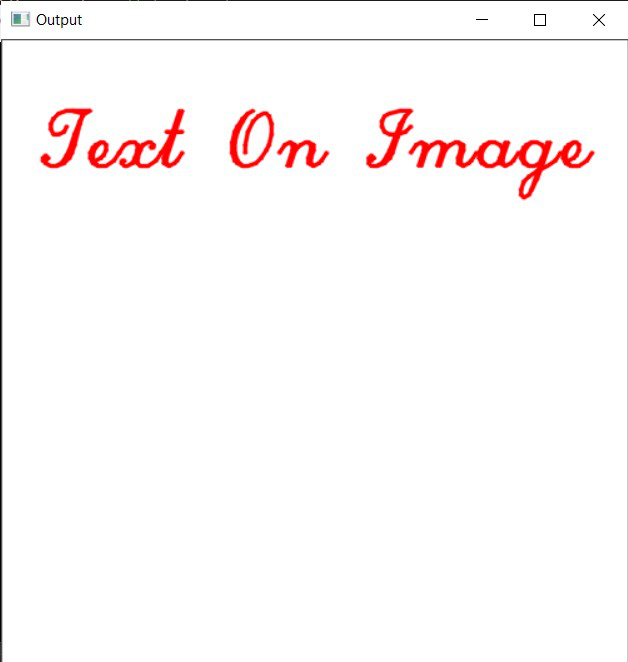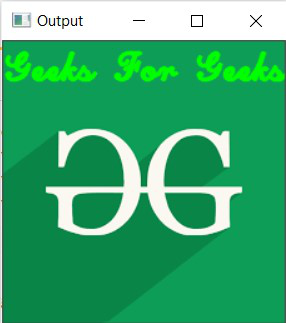# Write on an image using openCV in C++

• Last Updated : 04 Feb, 2021

In this article, we will discuss how to write over an image using OpenCV C++. Function putText() from OpenCV C++ library will be used to write text on an image.

Program 1:

Below program shows how to write text over a blank background image:

## C++

 `// C++ program to demonstrate the ` `// above approach ` `#include ` `#include ` ` `  `// Library to include for ` `// drawing shapes ` `#include ` `#include ` `using` `namespace` `cv; ` `using` `namespace` `std; ` ` `  `// Driver Code ` `int` `main(``int` `argc, ``char``** argv) ` `{ ` `    ``// Create a blank image of size ` `    ``// (500 x 500) with white background ` `    ``// (B, G, R) : (255, 255, 255) ` `    ``Mat image(500, 500, CV_8UC3, ` `              ``Scalar(255, 255, 255)); ` ` `  `    ``// Check if the image is created ` `    ``// successfully. ` `    ``if` `(!image.data) { ` `        ``cout << ``"Could not open or"` `             ``<< ``" find the image"` `             ``<< endl; ` `        ``return` `0; ` `    ``} ` ` `  `    ``// Writing over the Image ` `    ``Point org(30, 100); ` `    ``putText(image, ``"Text On Image"``, org, ` `            ``FONT_HERSHEY_SCRIPT_COMPLEX, 2.1, ` `            ``Scalar(0, 0, 255), 2, LINE_AA); ` ` `  `    ``// Show our image inside a window. ` `    ``imshow(``"Output"``, image); ` `    ``waitKey(0); ` ` `  `    ``return` `0; ` `} `

Output:Program 2:

Below program shows how to write text over a loaded image:

## C++

 `// C++ program to demonstrate the ` `// above approach ` `#include ` `#include ` ` `  `// Library to include for ` `// drawing shapes ` `#include ` `#include ` `using` `namespace` `cv; ` `using` `namespace` `std; ` ` `  `// Driver Code ` `int` `main(``int` `argc, ``char``** argv) ` `{ ` `    ``// Create a blank image of size ` `    ``// (500 x 500) with white background ` `    ``// (B, G, R) : (255, 255, 255) ` `    ``Mat image = imread(``"C:/Users/harsh/Downloads/geeks.png"``, ` `                       ``IMREAD_COLOR); ` ` `  `    ``// Check if the image is ` `    ``// created successfully. ` `    ``if` `(!image.data) { ` `        ``cout << ``"Could not open or"` `             ``<< ``" find the image"` `<< std::endl; ` `        ``return` `0; ` `    ``} ` ` `  `    ``// Writing over the Image ` `    ``Point org(1, 30); ` `    ``putText(image, ``"Geeks For Geeks"``, org, ` `            ``FONT_HERSHEY_SCRIPT_COMPLEX, 1.0, ` `            ``Scalar(0, 255, 0), 2, LINE_AA); ` ` `  `    ``// Show our image inside a window. ` `    ``imshow(``"Output"``, image); ` `    ``waitKey(0); ` ` `  `    ``return` `0; ` `} `

Output:My Personal Notes arrow_drop_up
Recommended Articles
Page :